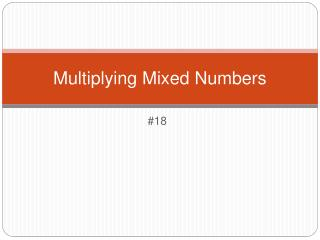DownloadDownload PresentationMultiplying Mixed Numbers

# Multiplying Mixed Numbers

Télécharger la présentation## Multiplying Mixed Numbers

- - - - - - - - - - - - - - - - - - - - - - - - - - - E N D - - - - - - - - - - - - - - - - - - - - - - - - - - -
##### Presentation Transcript

1. Remember! To write a mixed number as an improper fraction, start with the denominator, multiply by the whole number, and add the numerator. Use the same denominator. 1 1 • 5 + 1 6 __ _________ __ 5 5 5 = = 1

2. 1 1 __ __ 4 3 Example 1: Multiplying Fractions and Mixed Numbers Multiply. Write each answer in simplest form. • 1

3. 2 5 __ __ 3 6 Example 2 Multiply. Write each answer in simplest form. 2 •

4. 1 2 __ __ 4 9 Example 3 Multiply. Write each answer in simplest form. • 2

5. Remember! You can estimate the product to see whether your answer is reasonable.

6. 1 2 __ __ 7 3 Example 4: Multiplying Mixed Numbers Find each product. Write the answer in simplest form. 1 • 2

7. 1 3 __ __ 6 4 Example 5 Find each product. Write each answer in simplest form. 1 • 2

8. 1 2 __ __ 4 3 Example 6 Find each product. Write each answer in simplest form. 3 • 2

9. 2 1 __ __ 3 8 Example 7 Find each product. Write each answer in simplest form. 3 • 2